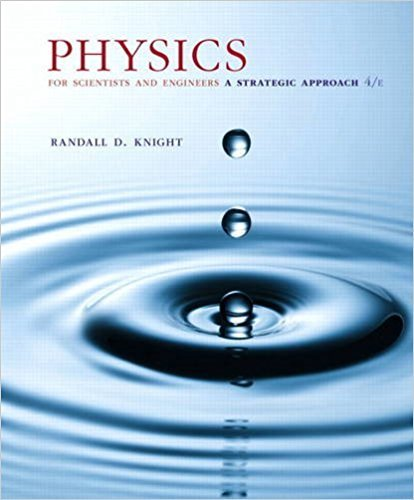×
×

# An infinite slab of charge of thickness 2z0 lies in theISBN: 9780134081496 191

## Solution for problem 24.73 Chapter 24

Physics for Scientists and Engineers: A Strategic Approach, Standard Edition (Chs 1-36) | 4th Edition

• Textbook Solutions
• 2901 Step-by-step solutions solved by professors and subject experts
• Get 24/7 help from StudySoup virtual teaching assistantsPhysics for Scientists and Engineers: A Strategic Approach, Standard Edition (Chs 1-36) | 4th Edition

4 5 0 327 Reviews
27
3
Problem 24.73

An infinite slab of charge of thickness 2z0 lies in the xyplane between z = -z0 and z = +z0. The volume charge density r 1C/m3 2 is a constant. a. Use Gausss law to find an expression for the electric field strength inside the slab 1-z0 z z02. b. Find an expression for the electric field strength above the slab 1z z02. c. Draw a graph of E from z = 0 to z = 3z0.

Step-by-Step Solution:
Step 1 of 3
Step 2 of 3

Step 3 of 3

##### ISBN: 9780134081496

The answer to “An infinite slab of charge of thickness 2z0 lies in the xyplane between z = -z0 and z = +z0. The volume charge density r 1C/m3 2 is a constant. a. Use Gausss law to find an expression for the electric field strength inside the slab 1-z0 z z02. b. Find an expression for the electric field strength above the slab 1z z02. c. Draw a graph of E from z = 0 to z = 3z0.” is broken down into a number of easy to follow steps, and 77 words. This textbook survival guide was created for the textbook: Physics for Scientists and Engineers: A Strategic Approach, Standard Edition (Chs 1-36), edition: 4. This full solution covers the following key subjects: . This expansive textbook survival guide covers 42 chapters, and 4463 solutions. The full step-by-step solution to problem: 24.73 from chapter: 24 was answered by , our top Physics solution expert on 12/28/17, 08:06PM. Physics for Scientists and Engineers: A Strategic Approach, Standard Edition (Chs 1-36) was written by and is associated to the ISBN: 9780134081496. Since the solution to 24.73 from 24 chapter was answered, more than 232 students have viewed the full step-by-step answer.

Unlock Textbook Solution